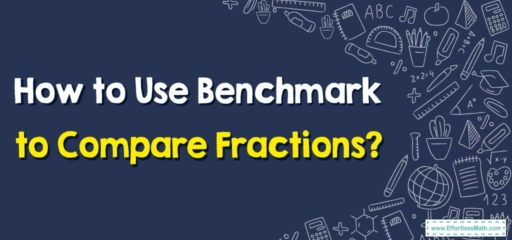# How to Use Benchmark to Compare Fractions?

A benchmark is a reference number that can be used to compare two fractions. Here you can learn more about using benchmarks to compare fractions.## A Step-by-step guide to using benchmarks to compare fractions

Definition of a Benchmark fraction: A common fraction utilized for comparing other fractions is called a benchmark fraction

These are simple common fractions everyone is accustomed to and allow seeing complicated fractions much simpler.

It’s possible to divide any object you want to measure easily or to compare it into two equal parts. So, the most common place benchmark fraction instance is (one-half).

You can also write in different formats or equivalent fractions, like, etc. Currently, one can do a comparison of the other fractions having different denominators to one half.

### Using Benchmark to Compare Fractions-Example:

Compare each fraction to the benchmark. $$\frac{4}{8}\:\left(\right)\:1$$, $$\frac{4}{3}\:\left(\right)\:1$$. Then, use this information to compare the two fractions. $$\frac{4}{8}\:\left(\right)\:\frac{4}{3}$$

Solution: First, compare each fraction to the benchmark.

$$\frac{4}{8}\:\left(<\right)\:1$$

$$\frac{4}{3}\:\left(>\right)\:1$$

Now, use this information to compare the two fractions.

$$\frac{4}{8}\:\left(<\right)\:\frac{4}{3}$$

## Exercises forUsing Benchmark to Compare Fractions

### Compare each fraction to the benchmark.Then compare the two fractions.

1. $$\color{blue}{\frac{5}{4}\:\left(\right)\:1,}$$ $$\color{blue}{\frac{5}{9}\:\left(\right)\:1, \frac{5}{9}\:\left(\right)\:\frac{5}{4}}$$
2. $$\color{blue}{\frac{2}{3}\:\left(\right)\:\frac{1}{2}, \frac{7}{15}\:\left(\right)\:\frac{1}{2}, \frac{2}{3}\:\left(\right)\:\frac{7}{15}}$$
1. $$\color{blue}{\frac{5}{4}\:\left(>\right)\:1,\frac{5}{9}\:\left(<\right)\:1,\frac{5}{4}\:\left(>\right)\:\frac{5}{9}}$$
2. $$\color{blue}{\frac{2}{3}\:\left(>\right)\:\frac{1}{2},\frac{7}{15}\:\left(<\right)\:\frac{1}{2}, \frac{2}{3}\:\left(>\right)\:\frac{7}{15}}$$

### What people say about "How to Use Benchmark to Compare Fractions? - Effortless Math: We Help Students Learn to LOVE Mathematics"?

No one replied yet.

X
30% OFF

Limited time only!

Save Over 30%

SAVE $5 It was$16.99 now it is \$11.99